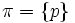# P-solvable group

The article defines a property of groups, where the definition may be in terms of a particular prime that serves as parameter
View other prime-parametrized group properties | View other group properties

## Definition

Let$G$ be a finite group and$p$ be a prime number. We say that$G$ is a p-solvable group if it satisfies the following equivalent conditions:

•$G$ has a subnormal series where all the quotients are either$p$-groups or have orders relatively prime to$p$.
•$G$ is a pi-separable group for$\pi = \{ p \}$. In other words,$G$ has a p-series.
• All the composition factors of$G$ that are non-abelian do not have$p$ dividing their order.

Note that if$p$ does not divide the order of$G$,$G$ is$p$-solvable. Further, a finite group is a finite solvable group if it is$p$-solvable for every prime$p$ dividing the order of$G$.

There is a notion of p-length to measure the length of a p-solvable group; briefly, it measures the number of the successive quotient groups that are p-groups in any$p$-series that minimizes this number.